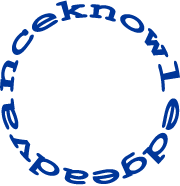# Character - Ring

Time Limit : 1 sec, Memory Limit : 131072 KB
Japanese version is here

# Ring

Write a program which finds a pattern $p$ in a ring shaped text $s$.## Input

In the first line, the text $s$ is given.
In the second line, the pattern $p$ is given.

## Output

If $p$ is in $s$, print Yes in a line, otherwise No.

## Constraints

• $1 \leq$ length of $p \leq$ length of $s \leq 100$
• $s$ and $p$ consists of lower-case letters

## Sample Input 1

vanceknowledgetoad


## Sample Output 1

Yes


## Sample Input 2

vanceknowledgetoad

No